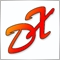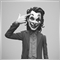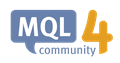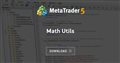# Lot Validation ?23290

hello,

need help please.

how to get validation to use lot = 1.0 or lot=1.10 ?

input lot = 1.02, error

input lot = 1.1, error

if I input manual Lot=1 or lot = 1.10, then passed.

But if I use script validation below, then get error 131

OrderSend error 131

```double cLOT(double ilot){

double ilot_max =MarketInfo(Symbol(),MODE_MAXLOT);
double ilot_min =MarketInfo(Symbol(),MODE_MINLOT);

int digit=0;
if(ilot_min==0.001) { digit=3; }
else if(ilot_min==0.01) { digit=2; }
else if(ilot_min==0.1) { digit=1; }

if(ilot>ilot_max) { ilot=ilot_max; }
if(ilot<ilot_min) { ilot=ilot_min; }

return(NormalizeDouble(ilot,digit));

}```

i use minstep, error also

```double cLOT(double ilot){

double ilot_max =MarketInfo(Symbol(),MODE_MAXLOT);
double ilot_min =MarketInfo(Symbol(),MODE_MINLOT);
double ilot_step =MarketInfo(Symbol(),MODE_MINSTEP);

int digit=0;
if(ilot_step==0.001) { digit=3; }
else if(ilot_step==0.01) { digit=2; }
else if(ilot_step==0.1) { digit=1; }

if(ilot>ilot_max) { ilot=ilot_max; }
if(ilot<ilot_min) { ilot=ilot_min; }

return(NormalizeDouble(ilot,digit));

}```

Thanks for help..28955

Siti Latifah:

hello,

need help please.

how to get validation to use lot = 1.0 or lot=1.10 ?

check against MODE_LOTSTEPMarketInfo() Identifiers - Appendixes - MQL4 Tutorial
• book.mql4.com
MarketInfo() Identifiers - Appendixes - MQL4 TutorialModerator
29897

Siti Latifah:

i use minstep, error also

`double ilot_step =MarketInfo(Symbol(),MODE_MINSTEP);`

What is MODE_MINSTEP?

Does your code compile?23290

Keith Watford:

What is MODE_MINSTEP?

Does your code compile?

sorry, I mean LotStep23290

Mohamad Zulhairi Baba:

check against MODE_LOTSTEP

yes.. thanks.. done4093

Try the function RoundVolume() in my Math Utils library

https://www.mql5.com/en/code/20822Math Utils
• www.mql5.com
Handy functions for comparison and rounding of floating-point numbers (prices, lots and money).